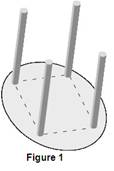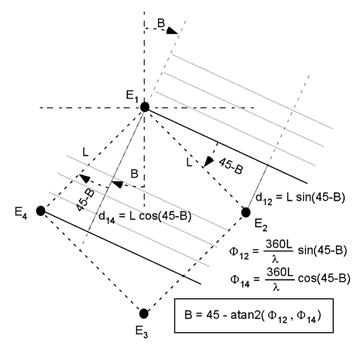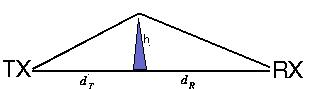# Bearing Determination with Quad Element Antennas

By Armando RodriguezThe quad element antenna refers to an array like the one shown in Figure 1. Elements are ¼l in length, the size of the square may vary for each design but is always less than l/2. The frequency for the TCAS receiver is 1090 MHz, so the wavelength l is 10.83”.

## Bearing Determination by Phase

The four element antenna is used by both Honeywell and Collins for intruder bearing detection in their phase TCAS systems.  The physics behind this detection principle is depicted in the following figure:Figure 2.

The phases in the above equations would be the ones that would be measured in opened elements, or in other words, in the unrealistic absence of currents. Yet, some energy needs to be absorbed from the incident wave to feed the receiver input circuits, so there will definitely be current in every element. These reactive currents will induce voltages in the neighboring elements affecting the phases to be measured. Fortunately, symmetry forces any reactive phase shift in F43 to be the same as in F12 and also forces shifts in F23 to be the same as in F14, So, as for canceling out the mentioned reactive effects of a real antenna, the sum of these phase shifts is introduced in the above expression for the bearing.

B = 45 – atan2(F12 + F43 , F14  + F23)                                                                     1

If we change the references so as to keep the numbering order we get:

B = 45 – atan2(F12 - F34 , F23  - F41)                                                                       2

Also the above can be expressed as:

B = atan2(F23  - F41 F12 - F34) - 45                                                                       3

It may be shocking that the expression for the bearing B is not dependent on the actual size of the antenna, yet it is not that just any size would the same; small antennas render smaller phase shifts for the same bearings. In other words, smaller antennas are less sensitive to intruder bearings changes than bigger ones. Let’s do some error propagation.

Allow Q to be the quotient in the inverse tangent

Q = Y/X = (F23  - F41)/(F12 - F34)                                                                                             4

Differentiating the quotient

dQ = dY/X – YdX/X2 = (Y/X)(dY/Y – dX/X) = Q(dX/X - dY/Y)                             5

The increments absolute values dX and dY may be taken as the unwanted RF path differences, while Y and X, are the phase spans. The signs of the unwanted differences must be assumed to render the worse case calculation, so let:

dQ = 2Q dA/A                                                                                                                    6

Now, differentiating the inverse tangent respect to Q:

dB = (180/p) dQ/(1 +Q2                                                                                               7

Combining 6 and 7:

dB = (180/p) (dA/A) 2Q/(1 +Q2)

The function 2Q/(1+ Q2) has a maximum of 1 for Q=1; the maximum for dB must then be:

dB = (180/p) (dA/A)                                                                                                        8

The maximum acceptable path error for a specified bearing tolerance dB is:

dA = dB A/(180/p)o                                                                                                         9

Say L = 3.8",since l =  1084" at 1090 MHz, then the maximum phase shift or phase span would be 126°. If  dB was to be specified to less than 10°, being A equal to its phase span of 126°, equation 9 would render that the maximum path error that could be tolerated is 22°,which would allow about half an inch error in the coaxial cabling lengths while in operation. A smaller antenna, say one with L = 2.65", would feature a phase span of only 88°. If a 3° accuracy is specified the path tolerance would only be 0.1". In this case, even small temperature gradients during flight may amount to these differences in thermal expansion, so periodic recalibration would be necessary.

## Bearing Determination by Amplitude

A perfectly loaded dipole absorbs power from an incident electro-magnetic wave, leaving a shadow behind it.  Though this shadowing effect practically disappears due to diffraction after a few wave lengths, a dipole within a shadow will be absorbing less power from the wave. The amplitudes of the received signals in quad dipole antenna will then depend on the wave angle of incidence, since the dipoles in the back will be shadowed more or less depending on how behind they are to the front ones. TCAS amplitude systems take advantage of this effect allowing their bearing determinations to challenge in accuracy those of phase systems.

For estimating just how much attenuation could be involved in this effect the front dipole may be simplified a perfectly opaque object with an area equal its effective area Aeff.

Aeff = G l2 /4p                                                                                                             1

Where G, is the Gain or directivity of the antenna that for the Hertzian dipole is 3/2. Considering this area to be shaped as a rectangle with a length equal to that of the half wave dipole, we get the following expression for it width W:

W = Aeff /(l /2) = 3l /4p                                                                                               2

Going now to the problem of the edge diffraction, consider a path profile model for the single knife edge diffraction*.Figure 3

If the direct line-of-sight is obstructed by a single knife-edge type of obstacle, with height h we define the following diffraction parameter v:

v  = h Ö((2/l)(1/dr+1/dR)                                                                                           3

Where dt and dR are the terminal distances from the knife edge. The diffraction loss, expressed in dB, can be closely approximated by

AD=0                                                                      if v < 0                                             4

AD=6 + 9 v + 1.27 v2                                          if 0 < v < 2.4                                        5

AD=13 + 20 log v                                                 if v >2.4                                               6

The attenuation over rounded obstacles is usually higher than AD in the above formulas. Since our object is not a sharp one, the actual attenuations can be expected to be greater that the calculations.

Since we are dealing with plane, waves the distance to the transmitter can be regarded as infinite; let dR be equal to ¼ l, which is about the size L in the antennas; finally, let h be equal to ½W:

v  = ½WÖ((2/l)(1/(¼ l)) = (W/l)Ö2= 3/(22) = 1.06                                               7

Substituting in equation 5: